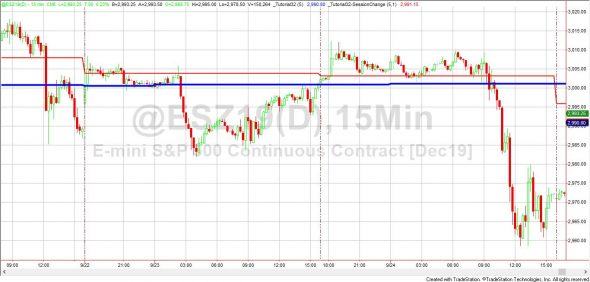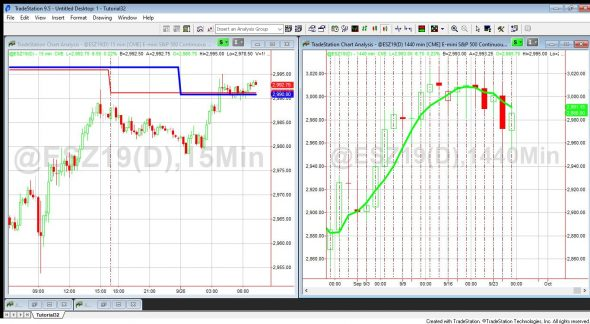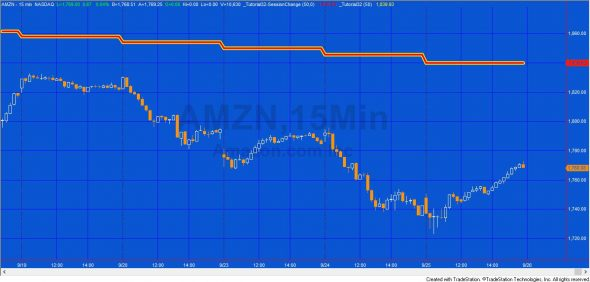# Tutorial 32a | Daily average on an intraday chart using either day or session breaks

Tutorial 32 calculates and plots a daily moving average on an intraday chart. The user has the option to calculate the average using either:

In the original tutorial 32 the moving average calculated differed from that calculated using the standard moving average indicator, applied to a daily or 1440 minute chart for a continuous futures contract where the session ended intraday. The main reason for this is that the moving average indicator’s calculations based on when a session is complete (rather than for each new day).

In addition, the close of a daily bar is the exchange provided settlement price, whereas the session close from intraday data is the last traded price.Bother the tutorial32 (blue line) and the tutorial 32a (red line) applied to a 15 minute @ESZ19 chart, displaying a 5 day moving average.

Tutorial 32a does not use the CloseD function (used in the original tutorial 32) but rather uses a dynamic array to store day or session close information in order to create a daily moving average on an intraday chart.To check the calculation, the standard moving average 1 line is applied to a 1440 minute chart. We compare the red line on the intraday chart with the value of the penultimate bar of the green line on the 1440 chart.

The unprotected program for TradeStation (version 8.7 and above) and MultiCharts is available for immediate download for \$39.95 here:

The ELD includes te new program (_Tutorial32A) and the original program (_Tutorial32).In this example a 50 moving average is plotted on a 15 minute AMZN chart. Both the tutorial 32 program (yellow line) and the tutorial 32a program (red line) are applied with the user input for Tutorial32a, DayOrSess, set to 0.
The number of days worth of data loaded on the chart must be greater than the user input: DaysBack. The user input: DayOrSess must be set to 0 or 1.